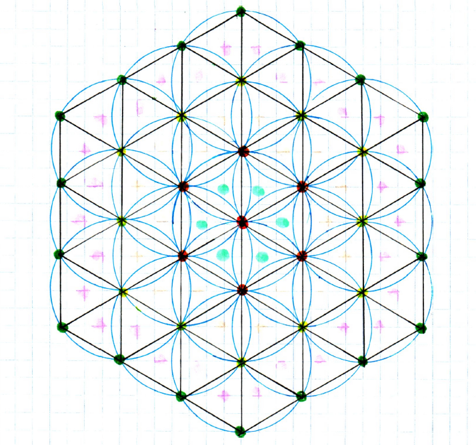# class full of students in the social distance world

These days, people from all over the world learn how to live with the covid-19 as part of our life. In my country we are back to school but need to keep 2 meters between one student to another. This reality makes me think about these questions: What is the minimum area required for a classroom with X students in it? And what is the most efficient shape for this classroom? This is my solution to these questions, but I would be grateful to hear your thoughts about it.

In attempt to find the most efficient shape I started thinking about a circle. If we will draw a circle with radius of 2m around a student, the closest other students have to be on this circle. We can put max 6 students on this circle to keep 2m between them. If we will continue and drew a circle (with radius of 2m) around each student and put 6 students on each circle (symmetrically located), we will get pattern like this- a hexagon. I think this equilateral hexagon is the most efficient shape.By drawing lines we can divide the hexagon to many equilateral triangles with side length of 2m, where the vertex represents students. Assume that we want to enter X students to an equilateral hexagon shape classroom.

N- the number of rings around the center (for example- at the image N=3)

How many students N rings can contain? the number of students at the 1st,2st,3st...N ring: 1,6,12,18...6N or 1, 6(1,2,3...N). the total number of students at N rings: X=1+6(1+2+3+...N). 1+2+3+...N = (1+N)N/2 so X=1 +3N(N +1) or X=3N^2 +3N +1 .

How many triangles there is in N rings? the number of tringles at the 1st,2st,3st...N ring: 6,18,30... . 6 is the first term and them each term is +12 to the previous one. the total number of triangles at N rings: Tri = N/2 * (12+12N-12) = 6N^2

the area of each triangle: A= √3 . the total area of N rings: area= Tri * A = 6√3 N^2 .

the connection between X (number of students) and N (number of rings): X=3N^2 +3N +1 or 3N^2 +3N +1 -X =0 . we need the positive solution of the quadratic equation- N= (√(12X-3) -3)/6

the connection between X (number of students) and area (the are of the hexagon class): area= 6√3 N^2 = 6√3 * [(√(12X-3) -3)/6]^2

after some algebra we get the final formula: area = 2√3 X -3√(4X-1) +1Note by Maya Toybenshlak
11 months ago

This discussion board is a place to discuss our Daily Challenges and the math and science related to those challenges. Explanations are more than just a solution — they should explain the steps and thinking strategies that you used to obtain the solution. Comments should further the discussion of math and science.

When posting on Brilliant:

• Use the emojis to react to an explanation, whether you're congratulating a job well done , or just really confused .
• Ask specific questions about the challenge or the steps in somebody's explanation. Well-posed questions can add a lot to the discussion, but posting "I don't understand!" doesn't help anyone.
• Try to contribute something new to the discussion, whether it is an extension, generalization or other idea related to the challenge.
• Stay on topic — we're all here to learn more about math and science, not to hear about your favorite get-rich-quick scheme or current world events.

MarkdownAppears as
*italics* or _italics_ italics
**bold** or __bold__ bold
- bulleted- list
• bulleted
• list
1. numbered2. list
1. numbered
2. list
Note: you must add a full line of space before and after lists for them to show up correctly
paragraph 1paragraph 2

paragraph 1

paragraph 2

[example link](https://brilliant.org)example link
> This is a quote
This is a quote
    # I indented these lines
# 4 spaces, and now they show
# up as a code block.

print "hello world"
# I indented these lines
# 4 spaces, and now they show
# up as a code block.

print "hello world"
MathAppears as
Remember to wrap math in $$ ... $$ or $ ... $ to ensure proper formatting.
2 \times 3 $2 \times 3$
2^{34} $2^{34}$
a_{i-1} $a_{i-1}$
\frac{2}{3} $\frac{2}{3}$
\sqrt{2} $\sqrt{2}$
\sum_{i=1}^3 $\sum_{i=1}^3$
\sin \theta $\sin \theta$
\boxed{123} $\boxed{123}$

## Comments

Sort by:

Top Newest

Well i guess the social distancing thing is getting worse day by day as the studies business assignment help are suffering a lot.

- 10 months, 3 weeks ago

Log in to reply

×

Problem Loading...

Note Loading...

Set Loading...